# How to Calculate the Amount of Soap to Fill A Soap Mold

Picture This:

Your new mold arrives in the mail and you are super excited to try it out with a new batch of soap. But then you wonder, will your favorite recipe fit into this mold? Will it be enough? Will it be too much? This mold looks a little bigger/smaller than your previous ones. What do you do?

I'm going to tell you exactly what you need to do. We're going to figure out how much oil we need for the recipe that will fill this mold. We're going to do some simple calculations, and from there you'll figure out the amount of oil. You'll then use this information to enter your values in a soap calculator to get the full recipe, including the amount of water and lye.

Obviously different molds will yield different amounts of soap and it's important for us to get the calculations right to get the right amount of soap batter for our mold.

If you're someone that gets a little anxious when it comes to math, don't worry, this is really simple.. Just follow along.

## 1. If Your Mold is Square or Rectangular

First we need to figure out the exact volume of the mold: You’re going to measure the length, width and depth of your mold to get the exact volume of Soap that you will make.  It’s important to measure the Inside of your mold for this part.  In the pictures below, you can see that I used a tape measure to measure the Length, Width, and Depth of my rectangular loaf mold.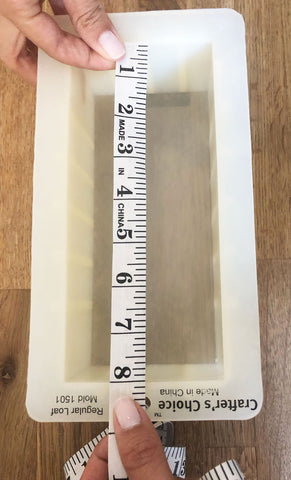The Length is 8 inches.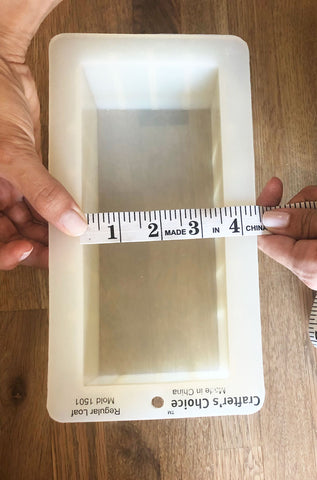The Width is 3.5 inches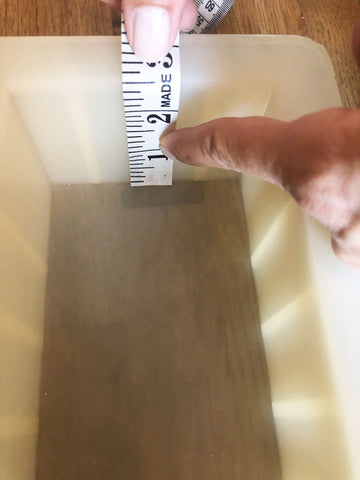The Depth is 2.5 inches.

(I'm not pointing with my index finger, I'm just holding the tape measure down. You can see that the tape measure reachers the top edge of the mold at 2.5 mark) - If you don't want to fill the mold to the very end, feel free to take the depth you want, for example 2.2 inches. But for this example, we're going to assume I'm making enough soap to fill my mold to the top.

Now to calculate the volume we follow the formula Length x Width x Depth

8" x 3.5" x 2.5" = 70 cubic inches which also means this mold can hold 70 oz of Soap.

But we need figure out how much OIL we need in our recipe and to do that we multiply the volume by 0.4 (or %40). Some soap makers multiply by 0.40 and some soapers multiply by 0.39. The idea is that the oils will make up about %40 of the total volume of the soap.

70 x 0.4 = 28 oz of Oil will be needed for the recipe to fill this mold

And if you like to work in grams like I do, you multiply the oz by 28.375

28oz x 28.375 = 794.5 gm of oil

Now that you have how much oil you need, you can enter the percentages of your recipe into a soap calculator and get the exact amount of each oil that you need for the recipe. We will take a look at this next week.

## 2. If you have a Circular or Cylinder Shaped Mold

If you have a circular or tube shaped mold you have to calculate the volume differently.

First: you will have to measure the diameter of the mold.

Second: Divide the diameter by 2 to get the radius.

Third: Measure the Length/Height of the mold.

To measure the cubic volume of a cylinder shaped mold you need to follow the following equations:

Pi  x radius squared x height of the mold.

Pi is a constant equal to 3.14

Height is the total length of the cylinder mold or the depth of a circular mold.

So to give you an example using the cylinder mold I have look at the pictures below: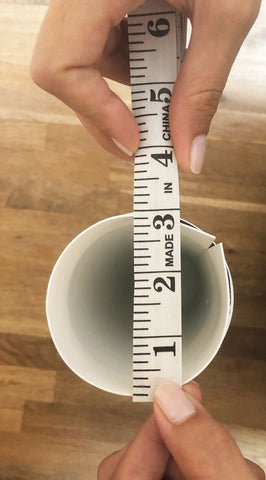I measured the diameter and found it to be about 3".

So the radius is 3 divided by 2 = 1.5".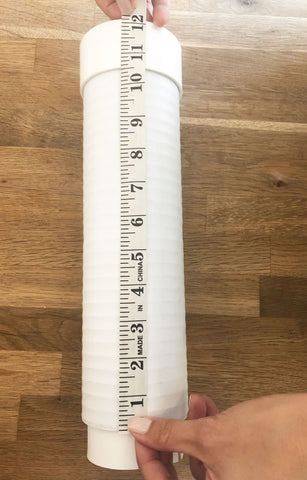The length of this tube is 12"

Now to plug in all this information:

The formula for measuring the cubic volume of a cylinder is

3.14 x 1.5 x 1.5 x 12 = 84.78 cubic inches

To calculate how much oil we need in our recipe, we multiply this number by 0.4

84.78 x 0.4 =33.912 .

So 33.912 oz is how much oil we need in our recipe.

To turn this value into grams because I prefer working in grams, we multiply this number by 28.375

33.912 x 28.375 = 962.253 gm of oil

I hope you found this article helpful.  You don't need to memorize anything. Feel free to come back to this blog post as many times as you like. And if you have any questions, remember, I'm only an email away.

Next week, we’ll talk about how to understand a recipe written in percentages and how it applies to measuring how much oil you need in the mold.

Until next week,

Sarah